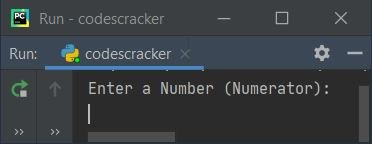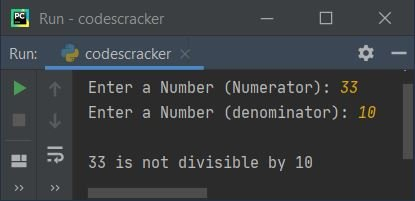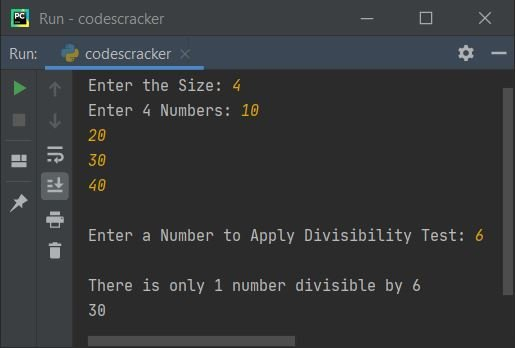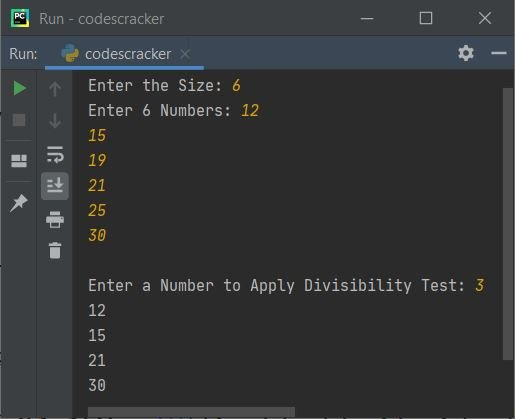# Python Program to Check Number is Divisible by Another Number

This article is created to cover some programs in Python, that checks whether a number is divisible by another number or not. Both numbers must be entered by user. Here are the list of programs:

• Check whether a Number is Divisible by Another Number or Not
• Divisibility Test with Multiple Numbers. That is, the program find and prints all the numbers from a list of numbers, that are divisible by a particular number
• Divisibility Test Program using user-defined Function

## Check a Number is Divisible by Another

This program checks whether a number (entered by user) is divisible by another number (also entered by user) or not. The question is, write a Python program to perform divisibility test. Here is its answer:

```print("Enter a Number (Numerator): ")
numn = int(input())
print("Enter a Number (denominator): ")
numd = int(input())

if numn%numd==0:
print("\n" +str(numn)+ " is divisible by " +str(numd))
else:
print("\n" +str(numn)+ " is not divisible by " +str(numd))```

Here is the initial output produced by this Python program:Now supply inputs say 21 as number, press `ENTER` key, then enter another number say 3 to check whether this number is divisible by 21 or not, like shown in the snapshot given below:Note - The str() converts into a string type value.

#### Modified Version of Previous Program

This is the modified version of previous program. This program handles invalid inputs. The end skips inserting an automatic newline.

```print("Enter a Number (Numerator): ", end="")
try:
numn = int(input())
print("Enter a Number (denominator): ", end="")
try:
numd = int(input())
if numn%numd==0:
print("\n" +str(numn)+ " is divisible by " +str(numd))
else:
print("\n" +str(numn)+ " is not divisible by " +str(numd))
except ValueError:
print("\nInvalid Input!")
except ValueError:
print("\nInvalid Input!")```

Here is its sample run with 33 and 10 as user inputs:## Divisibility Test with Multiple Numbers

Now this program performs divisibility test with multiple numbers at once. That is, the program receives the size, then receives numbers of given size. For example, if user enters 6 as size, then program further asks to enter six numbers, to store all these numbers in a list named nums. And finally receives a last input as a number to apply divisibility test like shown in the program given below:

```print("Enter the Size: ", end="")
tot = int(input())
print("Enter " +str(tot)+ " Numbers: ", end="")
nums = []
for i in range(tot):
nums.append(int(input()))

print("\nEnter a Number to Apply Divisibility Test: ", end="")
val = int(input())

res = []
for i in range(tot):
if nums[i]%val==0:
res.append(nums[i])

reslen = len(res)
if reslen>0:
if reslen>1:
print("\nThere are " +str(reslen)+ " numbers divisible by " +str(val))
for i in range(reslen):
print(res[i], end=" ")
print()
else:
print("\nThere is only 1 number divisible by " +str(val))
print(res)
else:
print("\nThere is no any number divisible by " +str(val))```

Here is its sample run with user inputs, 6 as size, 12, 15, 19, 21, 25, 30 as six numbers and then 3 as number to check for divisibility test, or to check 3 divides how many numbers from given six numbers like shown in the snapshot given below:Here is another sample run with user input 4 as size, 10, 20, 30, 40 as four numbers, and 6 as number to check its divisibility test:## Divisibility Test Program using Function

This program does the same job as of previous program. The only difference is, this is created using a user-defined function named divTest(). This function receives two numbers as its argument, and returns 1 if second number divides the first without leaving any remainder (or with leaving remainder as 0):

```def divTest(x, y):
if x%y==0:
return 1

print("Enter the Size: ", end="")
tot = int(input())

print("Enter " +str(tot)+ " Numbers: ", end="")
nums = []
for i in range(tot):
nums.append(int(input()))

print("\nEnter a Number to Apply Divisibility Test: ", end="")
val = int(input())

for i in range(tot):
if divTest(nums[i], val) == 1:
print(nums[i])```

Here is its sample run with same user input as of previous program's first sample run:Python Online Test

« Previous Program Next Program »